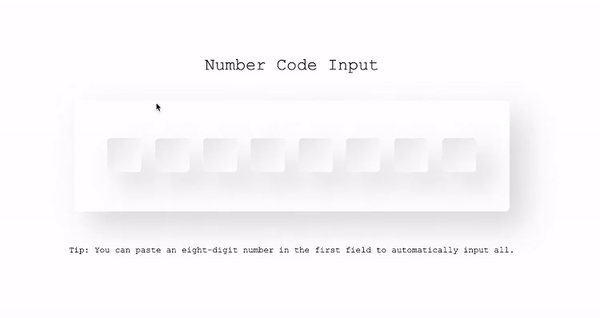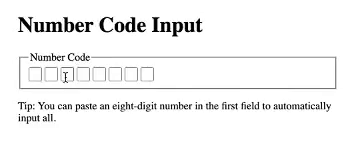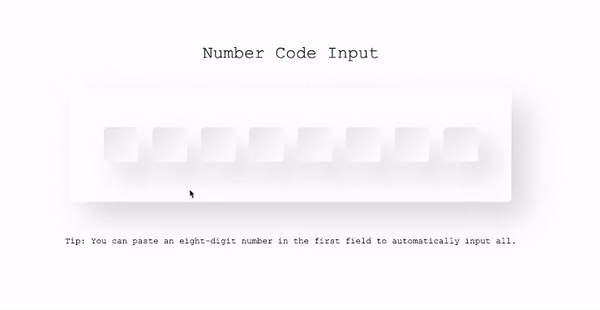Article

# Building a Number Code InputI often come across Number Code Inputs on various websites used for security measures, voucher codes and more. These Number Code Inputs often have a bit more functionality behind them then you might think at first. Let's create our own as an exercise.Number Code Input As you can see, the core functionality of these inputs is to enter one number at a time. The building of that happy flow can be quite easy to do. When playing around we can see that there a quite a few more functionalities. Let's first set the requirements: The Number Code Input should:

• automatically move to the next and stop at the last number input
• navigate from left to right with the arrow keys
• delete a single value in input and automatically going back to and delete the value of the previous input when hitting the backspace key
• delete the value of a focussed and previous input if backspace is pressed multiple times
• handle the pasting of several/all numbers in a number input You see, this is quite a bit of functionality. As always, I will build this custom input in a progressively enhanced way on three levels. Please read more about this in my blog "Writing progressively enhanced custom inputs for the web".

## Structural (HTML)Number Code Input with HTML
``````<fieldset name='number-code' data-number-code-form>
<legend>Number Code</legend>

<input type="number" min='0' max='9' name='number-code-0' data-number-code-input='0' required />
<input type="number" min='0' max='9' name='number-code-1' data-number-code-input='1' required />
<input type="number" min='0' max='9' name='number-code-2' data-number-code-input='2' required />
<input type="number" min='0' max='9' name='number-code-3' data-number-code-input='3' required />
<input type="number" min='0' max='9' name='number-code-4' data-number-code-input='4' required />
<input type="number" min='0' max='9' name='number-code-5' data-number-code-input='5' required />
<input type="number" min='0' max='9' name='number-code-6' data-number-code-input='6' required />
<input type="number" min='0' max='9' name='number-code-7' data-number-code-input='7' required />
</fieldset>
``````

Let's start with the structure. As you might have read in the article I mentioned, I need to provide a working HTML version first. The Number Code Input will consist of a fieldset containing all the separate number inputs. The fieldset is used to indicate that these inputs belong together. With the `legend`, I indicate what this group is. The inputs itself have a few needed attributes. Firstly, I declared that the input type must be a number. This is not just for validation but triggers the number keyboard layout on devices. This increases the user experience. Next to that, I've added a `min` value of 0 and a `max` value of 9. I did this so you can only enter a single number character per input. You might see some attributes like `data-number-code-input`. This has no function right now but will be used later on by the JavaScript. Great, the Number Code Input now allows for numbers being entered by the user. Let's proceed to the next step.

## Presentational (CSS)Number Code Input with HTML and CSS

``````legend {
font-size: 0;
}

input::-webkit-outer-spin-button, input::-webkit-inner-spin-button {
-webkit-appearance: none;
margin: 0;
}

input[type=number] {
-moz-appearance: textfield;
}
``````

Naturally, the style of the inputs is up to you. I did add a few things, besides nice visuals, to make the form a bit better. I set the `font-size` of the legend to 0 as visually we don't need it, but for screenreaders, we want to have that information available. Next to that, I hid the arrows in the input field as I did not find it needed in my use case. This is, of course, up to you. The Number Code Input is starting to look a lot better. Now let's add some interactivity.

## Behavioural (JavaScript)Number Code Input with HTML, CSS and JS Let's take the behavioural layer one requirement at a time.

### Automatically move to the next and stop at the last number input

I need to add some logic to call focus on the next number input if the case that the current number input is not the last one

``````const numberCodeForm = document.querySelector('[data-number-code-form]');
const numberCodeInputs = [...numberCodeForm.querySelectorAll('[data-number-code-input]')];

const handleInput = ({target}) => {
let currentIndex = Number(target.dataset.numberCodeInput);
const nextIndex = currentIndex + 1;

if(nextIndex < numberCodeInputs.length) {
numberCodeInputs[nextIndex].focus();
}
});
``````

I need to add an event listener that triggers when a key is pressed. I then focus on the next or previous number input if there is one.

``````const numberCodeForm = document.querySelector('[data-number-code-form]');
const numberCodeInputs = [...numberCodeForm.querySelectorAll('[data-number-code-input]')];

const handleKeyDown = e => {
const {code, target} = e;

const currentIndex = Number(target.dataset.numberCodeInput);
const previousIndex = currentIndex - 1;
const nextIndex = currentIndex + 1;

const hasPreviousIndex = previousIndex >= 0;
const hasNextIndex = nextIndex <= numberCodeInputs.length - 1

switch(code) {
case 'ArrowLeft':
case 'ArrowUp':
if (hasPreviousIndex) {
numberCodeInputs[previousIndex].focus();
}
e.preventDefault();
break;

case 'ArrowRight':
case 'ArrowDown':
if (hasNextIndex) {
numberCodeInputs[nextIndex].focus();
}
e.preventDefault();
break;

default:
break;
}
}

``````

### Delete a single value in an input and automatically going back to and delete the value of the previous input when hitting the backspace key

This functionality is pretty straight forward. I delete the value of the focussed number input and focus the previous number input if present.

``````const numberCodeForm = document.querySelector('[data-number-code-form]');
const numberCodeInputs = [...numberCodeForm.querySelectorAll('[data-number-code-input]')];

const handleKeyDown = e => {
const {code, target} = e;

const currentIndex = Number(target.dataset.numberCodeInput);
const previousIndex = currentIndex - 1;
const nextIndex = currentIndex + 1;

const hasPreviousIndex = previousIndex >= 0;
const hasNextIndex = nextIndex <= numberCodeInputs.length - 1

switch(code) {
case 'Backspace':
if (!e.target.value.length && hasPreviousIndex) {
numberCodeInputs[previousIndex].value = null;
numberCodeInputs[previousIndex].focus();
}
break;
default:
break;
}
}

``````

### Handle the pasting of several/all numbers in a number input

Finally, when pasting a value of multiple characters I want to automatically fill out the number input based on that value. This is important as this is a common way people fill out these forms.

``````const numberCodeForm = document.querySelector('[data-number-code-form]');
const numberCodeInputs = [...numberCodeForm.querySelectorAll('[data-number-code-input]')];

const handleInput = ({target}) => {
const inputLength = target.value.length;
let currentIndex = Number(target.dataset.numberCodeInput);

if (inputLength > 1) {
const inputValues = target.value.split('');

inputValues.forEach((value, valueIndex) => {
const nextValueIndex = currentIndex + valueIndex;

if (nextValueIndex >= numberCodeInputs.length) { return; }

numberCodeInputs[nextValueIndex].value = value;
});

currentIndex += inputValues.length - 2;
}

const nextIndex = currentIndex + 1;
});
``````

## Looking back

That's it. I build a progressively enhanced Number Code Input that deals with the several requirements we set. Feel free to have a look over at CodePen to play around with the Number Code Input and look further into the source code.